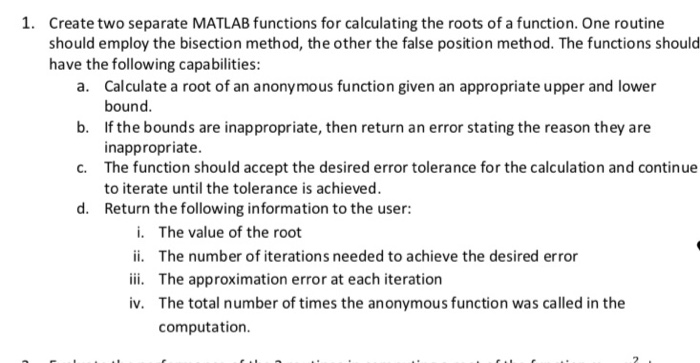Question

Need help programming if you can write the program outcompletely neatly that would help too1. Create two separate MATLAB functions for calculating the roots of a function. One routine should employ the bisection method, the other the false position method. The functions should have the following capabilities: a. Calculate a root of an anonymous function given